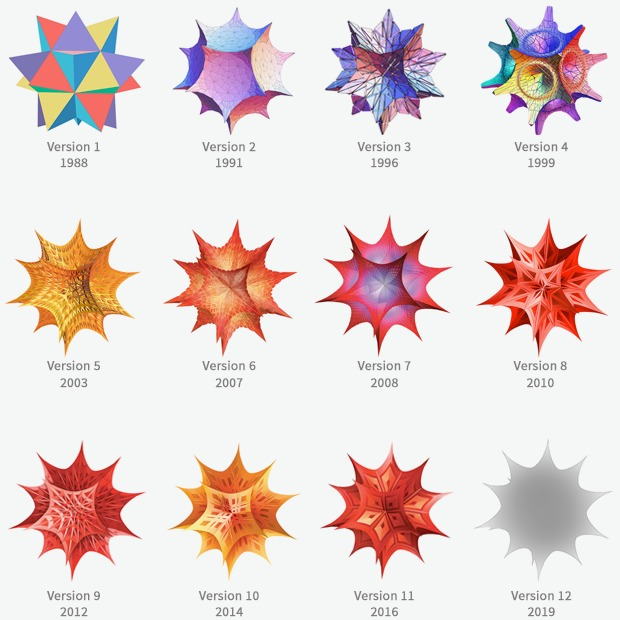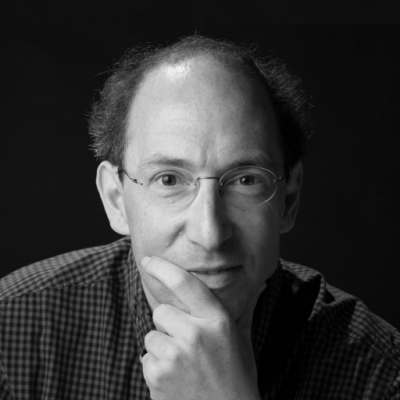# Mathematics & machines

### 27 Sep 2021 | 5:00pm

Conrad Wolfram describes how two brothers harnessed machines to do mathematics, changing the way we think about computational thinking.

Are machines intelligent? Far from approaching artificial general intelligence, AI seems not to have progressed beyond high-dimensional curve-fitting. But hiding in plain sight is perhaps the most compelling of all AI achievements: Mathematica, a general system for solving mathematical equations and manipulating mathematical expressions. Mathematica has developed as well into a functional programming language and the leading platform for visualising mathematics and data.

In this event, Conrad Wolfram tells the story of how he and his brother Stephen Wolfram transformed the way that scientists do mathematics. As well as giving an inside account of the past, Mr Wolfram also peers into the future. Can we use machines to help identify new mathematical conjectures and speed up theoretical research? Is computational thinking at the heart of scientific research, and even how we understand and teach mathematics? This seminar takes place in what used to be Michael Faraday’s private apartment, marking the launch of the London Institute’s seminar series in its new home at the Royal Institution in Mayfair.## Speakers